# Electronics and Communication Engineering - Electromagnetic Field Theory

6.

∇ .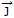= 0 is frequently known as

 A. continuity equation for steady currents B. Laplace equation C. Poisson's equation D. none of the above

Explanation:

Equation of continuity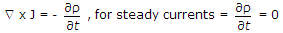∇ x J = 0.

7.

A rhombic antenna is a

 A. resonant antenna B. non-resonant antenna C. coupled antenna D. none of the above

Explanation:

No answer description available for this question. Let us discuss.

8.

Which of the following relations is valid?

 A. ∇ x ∇ x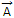= ∇(∇ .) - ∇2 A B. ∇ x ∇ x= ∇(∇ x) - ∇2 A C. ∇ x ∇ x= ∇2 A - (∇) D. none of the above

Explanation:

No answer description available for this question. Let us discuss.

9.

Diffraction of electromagnetic waves

 A. may occur around the edge of a sharp obstacle B. is caused by reflections from the ground C. arises only with the spherical wave fronts D. will occur when the waves pass through a large slot

Explanation:

No answer description available for this question. Let us discuss.

10.

The magnetic field intensity vector of a plane wave is given by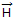(x, y, z, t) = 10 sin (5000t + 0.004x + 30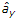), wheredenotes unit vector in y direction the wave is propagating with a phase velocity.

 A. 5 x 104 m/sec B. -3 x 108 m/sec C. -1.25 x 107 m/sec D. 3 x 108 m/sec

Explanation:(x, y, z, t) = 10 sin (5000t + 0.004x + 30)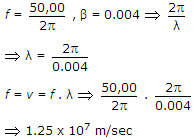Wave is propagating in - ve direction, hence

v = - 1.25 x 107 m/sec.